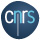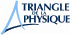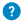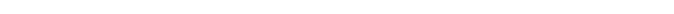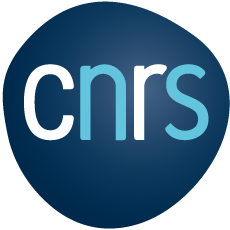Quantum measure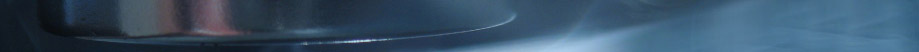Quantum mesure

All is probabilities

In quantum physics, since a particle is also a wave, what happens when you measure its position? During measurement, the interaction between the large size of the measurement tool and the small size of the quantum object makes the wavefunction of the object suddenly collapse at one particular spot. The experimenter perceives he is measuring a particle. But how does the wave “choose” where to collapse? In fact, the reduction can happen wherever the wave is present, but with different probabilities, depending on the value of the wavefunction. For instance, if the wavefunction has a bell shape, you will more likely find the corresponding particle in the centre of the bell rather than on the edges.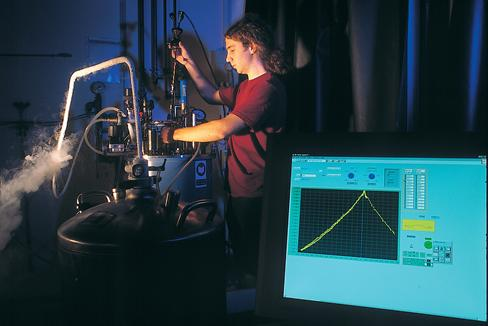After the quantum object is "collapsed" while being measured, it will go back to its wave-particle dual state until the following measurement. The measurement has hence affected the wave and forced the quantum object to start over in a new state. This differentiates quantum physics from classical physics where the experimenter does not have an impact on what he measures. Another weird thing in quantum measurements comes from the uncertainty principle, discovered by Heisenberg in 1927. According to this principle, one cannot precisely know at the same time both the momentum (mass times speed) and the position of an object. This principle is inviolable; if you measure one of these characteristics precisely, you cannot measure the other one.

The uncertainty principle is linked to the basics of quantum theory. A schematic way to understand this is to consider the wavefunction of the object to be measured. The momentum (mass times speed) is the inverse of the wavelength (the distance between two peaks). If the wave spreads across a large area, you can observe several periods, and it is then easy to precisely measure the wavelength, and, thus, the momentum. But you do not really know where the object is because the wave is spread. Conversely, if you know exactly where the particle is, the wave will be localized and it will be difficult to measure its period, and thus its momentum.